# Shear Stress Equation clearly explained - Average, Beam, Impact, Fluids shear stress

Did you realise that when you rub your hands together while washing, you are causing shear stress on the surface of your hands? Or that when you brush your teeth, there is shear stress on the surface of your teeth? In this article, we’ll discuss some examples of various shear stress equations.

## What are the basics of shear stress?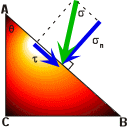Fig.1: Shear stress (τ) and normal stress (sn) acting on a line segment AB

Whenever two materials rub against or slide over each other, there is shear. While normal stress results from the force applied perpendicular to the surface of a material, shear stress occurs when force is applied parallel to the surface of the material. A few common everyday examples include the cutting of paper with scissors, rubbing our hands while washing, brushing our teeth, rubbing sandpaper on a  surface for polishing etc.

Fig.1 illustrates the shear stress (τ) and normal stress (sn) acting on a line segment AB.

Shear stress differs across materials and cross-sections and is measured using a set of formulas called the shear stress equations.

## Why are shear stress equations necessary?

Shear stress occurs whenever there is contact between two materials or components. Examples include stress exerted on a set of cantilever beams (with or without adhesion between layers), horizontal beams used in construction, pipelines carrying flowing fluids, soil when it is subjected to loads from the top surface etc. Shear stress equations help measure shear stress in different materials (beams, fluids etc.) and cross-sections, which play an important part in the design of engineering structures, to determine the load that can be carried. Most engineering structures are designed for both normal stress and shear stress limits.

## Shear stress equation (average)

General shear stress, represented by the Greek letter tau, τ, is given by the ratio of force applied to the area on which it acts.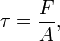Where,

• τ = shear stress
• F = force applied
• A = cross-sectional area of the material

Notes:

• Shear stress is the same irrespective of the direction in which it occurs, i.e., left to right or right to left.
• The above formula gives the average shear stress. In practical applications, shear stress is seldom uniform throughout the surface.

## Beam shear stress equation

Beam Shear is the internal shear stress that occurs on a beam when it is subjected to a shear force.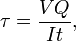Where,

• τ = beam shear stress
• V = shear force
• Q = static moment of the area (which is the summation of all areas multiplied by the distance from a particular axis)
• I = second area moment of the cross-section
• t = thickness of the material

## Impact shear stress equation

This gives the maximum shear stress in a solid round rod subjected to a force.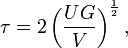Where,

• τ = impact shear
• U = kinetic energy, given as the sum of rotating kinetic energy and applied kinetic energy
• G = shear modulus/modulus of rigidity
• V = volume of the rod

## Fluids shear stress equation

Any fluid moving along a solid boundary will cause shear stress on the solid boundary. The shear stress for a Newtonian fluid, at a point y, is given by: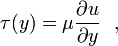Where,

• μ = dynamic viscosity of the fluid
• u = velocity of the flow along the boundary
• y = height above the boundary

## Wall Shear Stress

Following the above examples of shear stress equations, wall shear stress is the measure of the tangential component of the force exerted on a wall by a fluid flowing on its surface. Wall shear stress measurements are an important part of fluid mechanics. For instance, they could be key in understanding phenomena like corrosion, or the formation of deposits in pipes etc. The velocity gradient close to the wall is used to measure wall shear stress.

Depending on the system in which they are used, there are various techniques to measure wall shear stress. They can be broadly classified as follows:

1. Techniques, which use normal stress that is related to the shear stress – Stanton Gauge, K-Tube, Preston Tube, and sublayer fence.
2. Using a floating section of the surface to make a direct measurement of the shear stress through magnetic techniques or strain gauges.
3. Methods that rely on heat transfer or mass transfer. For mass transfer, an electrochemical reaction on a probe mounted on a surface produces an electrolysis current, whose measurement is directly related to shear stress. In the case of heat transfer, a probe is maintained at a specific temperature higher than its surroundings; the voltage thus used is related directly to shear stress.

## Conclusion

Shear stress occurs almost everywhere around us. More so in engineering structures, which comprise of a variety of components that are subjected to different kinds of loads. The measurement of shear stress, using the shear stress equations, thus forms an integral part of the design of these structures.

• Why are shear stress equations necessary?
• This article looks at some examples of shear stress equations: average shear stress, beam shear, impact shear and shear stress in fluids
• We'll talk about the measurement of wall shear stress in applications involving fluids.